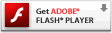# Plotting multiple lines on a figure in MATLAB

This MATLAB graphics tutorial shows you how you can plot multiple lines on the same plot.|